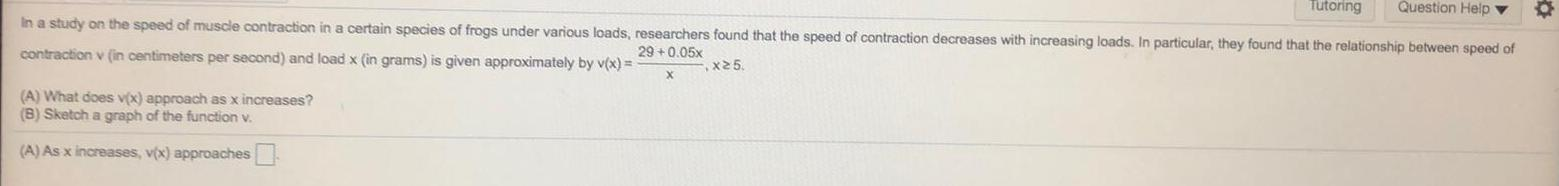Question:

# In a study on the speed of muscle contraction in a certain

Last updated: 7/21/2022In a study on the speed of muscle contraction in a certain species of frogs under various loads, researchers found that the speed of contraction decreases with increasing loads. In particular, they found that the relationship between speed of contraction v (in centimeters per second) and load x (in grams) is given approximately by v(x) = 29+0.05x/x, x≥5 (A) What does v(x) approach as x increases? (B) Sketch a graph of the function v. (A) As x increases, v(x) approaches